# RS Aggarwal Class 9 Maths Chapter-1 Real Numbers Solutions

## Access Solutions to RS Aggarwal Mathematics For Class 9 Chapter 1 Real Numbers Exercises 1A to 1F:

### RS Aggarwal Class 9 Mathematics Solutions Chapter 1 Real Numbers Exercise 1A

Question 1.
Solution:
A number in the form of pq where p and q are integers and q ≠ 0, is called a rational numberare all rational numbers.

Question 2.
Solution:
The given rational number are represented on a number line on given below :Question 3.
Solution:
We know that, if a and b are two rational numbers, then a rational number between a and b will beQuestion 4.
Solution:
Here, n = 3, x = 15, y = 14Question 5.
Solution:
Here, n=5, x = 25, y = 34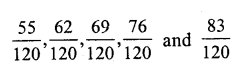Question 6.
Solution:
Here, n = 6, x = 3, y = 4Question 7.
Solution:
Here, n = 16, x = 2.1, y = 2.2### RS Aggarwal Class 9 Mathematics Solutions Chapter 1 Real Numbers Exercise 1B

Question 1.
Solution:
We know that a fraction pq is terminating if prime factors of q are 2 and 5 only.
Hence.
(i) 1380 and 16125 are the terminating decimals.

Question 2.
Solution: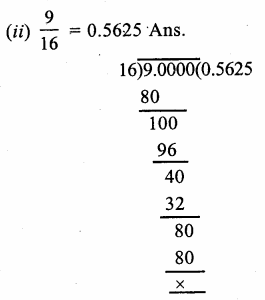Question 3.
Solution:
(i) Let,x = 0.3¯¯¯ = 0.3333…(i)
Then, 10x = 3.3333….Question 4.
Solution:
(i) True, because set of natural numbers is a subset of whole number.
(ii) False, because the number 0 does not belong to the set of natural numbers.
(iii) True, because a set of integers is a subset of a rational numbers.
(iv) False, because the set of rational numbers is not a subset of whole numbers.
(v) True, because rational number can be expressed as terminating or repeating decimals.
(vi) True, because every rational number can be express as repeating decimals.
(vii) True, because 0 = 01, which is a rational number Ans.

### RS Aggarwal Class 9 Mathematics Solutions Chapter 1 Real Numbers Exercise 1C

Question 1.
Solution:
Irrational numbers : Numbers which are not rational numbers, are called irrational numbers. Rational numbers can be expressed in terminating decimals or repeating decimals while irrational number can’t.
12 , 23 , 75 etc.are rational numbers and π, √2, √3, √5, √6….etc are irrational numbers

Question 2.
Solution:
(i) √4 = ±2, it is a rational number
(ii) √196 = ±14 it is a rational number
(iii) √21 It is irrational number.
(iv) √43 It is irrational number.
(v) 3 + √3 It is irrational number because sum of a rational and an irrational number is irrational
(vi) √7 – 2 It is irrational number because difference of a rational and irrational number is irrational
(vii) 23√6 . It is irrational number because product of a rational and an irrational number is an irrational number.
(viii) 0.6¯¯¯ = 0.6666…. It is rational number because it is a repeating decimal.
(ix) 1.232332333…. It is irrational number because it not repeating decimal
(x) 3.040040004…. It is irrational number because it is not repeating decimal.
(xi) 3.2576 It is rational number because it is a terminating decimal.
(xii) 2.3565656…. = 2.3 56¯¯¯¯¯ It is rational number because it is a repeating decimal.
(xiii) π It is an irrational number
(xiv) 227. It is a rational number which is in form of pq Ans.

Question 3.
Solution:
(i) Let X’OX be a horizontal line, taken as the x-axis and let O be the origin. Let O represent 0.
Taken OA = 1 unit and draw AB ⊥ OA such that AB = 1 unit. Join OB, Then,Question 4.
Solution:
Firstly we represent √5 on the real line X’OX. Then we will find √6 and √7 on that real line.
Now, draw a horizontal line X’OX, taken as x-axis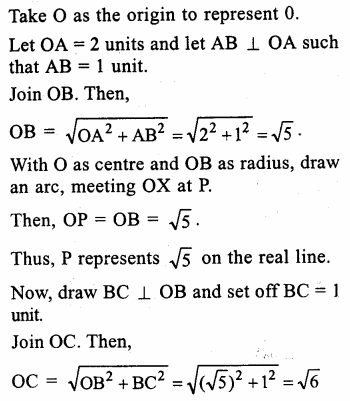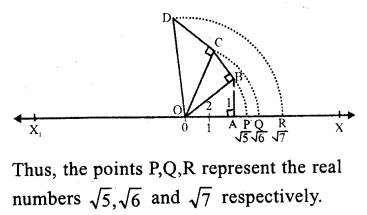Question 5.
Solution:
(i) 4 + √5 : It is irrational number because in it, 4 is a rational number and √5 is irrational and sum of a rational and an irrational is also an irrational.
(ii) (-3 + √6) It is irrational number because in it, -3 is a rational and √6 is irrational and sum or difference of a rational and irrational is an irrational.
(iii) 5√7 : It is irrational because 5 is rational and √7 is irrational and product of a rational and an irrational is an irrational.
(iv) -3√8 : It is irrational because -3 is a rational and √8 is an irrational and product of a rational and an irrational is also an irrational.
(v) 25 It is irrational because 2 is a rational and √5 is an irrational and quotient of a rational and an irrational is also an irrational.
(vi) 43 It is irrational because 4 is a rational and √3 is an irrational number and quotient of a rational and irrational is also an irrational.

Question 6.
Solution:
(i) True.
(ii) False, as the sum of two irrational number is irrational is not always true.
(iii) True.
(iv) False, as the product of two irrational numbers is irrational is not always true.
(v) True.
(vi) True.
(vii) False as a real number can be either rational or irrational.

### RS Aggarwal Class 9 Mathematics Solutions Chapter 1 Real Numbers Exercise 1D

Question 1.
Solution:Question 2.
Solution:Question 3.
Solution:Question 4.
Solution:Question 5.
Solution:
(i) Draw a line segment AB = 3.2 units (cm) and extend it to C such that BC = 1 unit.(ii) Find the mid-point O of AC.
(iii) With centre O and OA as radius draw a semicircle on AC
(iv) Draw BD ⊥ AC meeting the semicircle at D.
(v) Join BD which is √3.2 units.
(vi) With centre B and radius BD, draw an arc meeting AC when produced at E.
Then BE = BD = √3.2 units. Ans.

Question 6.
Solution:
(i) Draw a line segment AB = 7.28 units and produce is to C such that BC = 1 unit (cm)(ii) Find the mid-point O of AC.
(iii) With centre O and radius OA, draw a semicircle on AC.
(iv) Draw a perpendicular BD at AC meeting the semicircle at D
Then BD = √7.28 units.
(v) With centre B and radius BD, draw an arc which meet AC produced at E.
Then BE = BD = √7.28 units.

Question 7.
Solution:
(i) Closure property: The sum of two real numbers is always a real number.
(ii) Associative Law : (a + b) + c = a + (b + c), for all values of a, b and c.
(iii) Commutative Law : a + b = b + a for all real values of a and b.
(iv) Existance of Additive Identity : 0 is the real number such that: 0 + a = a + 0 = afor every real value of a.
(v) Existance of addtive inverse : For each real value of a, there exists a real value (-a) such that a + (-a) = (-a) + a = 0, Then (a) and (-a) are called the additive inverse of each other.
(v) Existence of Multiplicative Inverse. For each non zero real number a, there exists a real number 1a such that a . 1a = 1a . a = 1
a and 1a are called multiplicative inverse or reciprocal of each other.
(B) Multiplication
(i) Closure property: The product of two real numbers is always a real number.
(ii) Associative law : ab(c) = a(bc) for all real values of a, b and c
(iii) Commutative law : ab=ba for all real numbers a and b
(iv) Existance of Multiplicative Identity: clearly it is a real number such that 1.a = a.1 = a for every value of a.

### RS Aggarwal Class 9 Mathematics Solutions Chapter 1 Real Numbers Exercise 1E

Rationalise the denominator of each of the followings :

Question 1.
Solution:
Here,RF of √7 is √7Question 2.
Solution:
Here RF √3 is √3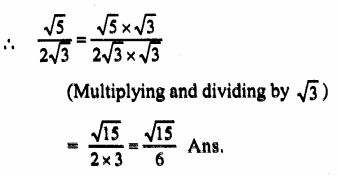Question 3.
Solution:
Here RF of 1(2+3) is 1(23)Question 4.
Solution:
Here RF is √5 + 2Question 5.
Solution:
Here RF is 5 – 3√2Question 6.
Solution:
Here RF is √6 + √5Question 7.
Solution:
Here RF = √7 – √3Question 8.
Solution:
Here RF = √3 – 1Question 9.
Solution:
Here RF = (3-2√2)Find the values of a and b in each of the following :

Question 10.
Solution:
3+131, RF = √3+1
(Multiplying and dividing by √3+1)Question 11.
Solution:
3+232, RF is 3+√2
(Multiplying and dividing by 3+√2)Question 12.
Solution:
In 565+6, RF is (5-√6)
(Multiplying and dividing by 5-√6)Question 13.
Solution:
In 5+237+43, RF is 7-4√3
(Multiplying and dividing by 7-4√3)Question 14.
Solution:Question 15.
Solution:Question 16.
Solution:
x = (4-√15)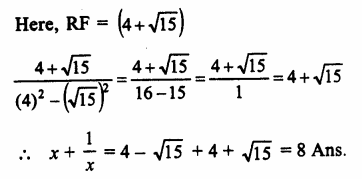Question 17.
Solution:
x = 2+√3Question 18.
Solution:### RS Aggarwal Class 9 Mathematics Solutions Chapter 1 Real Numbers Exercise 1F

Question 1.
Solution:
We know that
ap x aq = ap+q
∴ ThereforeQuestion 2.
Solution:
We know that
ap ÷ aq = ap-q
ThereforeQuestion 3.
Solution:
We know that
ap x bp = (ab)p
ThereforeQuestion 4.
Solution:
We know that
(ap)q =apqQuestion 5.
Solution:Question 6.
Solution:Question 7.
Solution:Access More:

Class: 6 Class: 7 Class: 8 Class: 9 Class: 10
Maths Maths Maths Maths Maths
Science Science Science Science Science
Social Science Social Science Social Science Social Science Social Science
English English English English English
Hindi Hindi Hindi Hindi Hindi
Extra Subjects Extra Subjects Extra Subjects Extra Subjects Extra Subjects